Main Content

## Create an FIR Filter Using Integer Coefficients

This section provides an example of how you can create a filter with integer coefficients. In this example, a raised-cosine filter with floating-point coefficients is created, and the filter coefficients are then converted to integers.

### Define the Filter Coefficients

To illustrate the concepts of using integers with fixed-point filters, this example will use a raised-cosine filter:

`b = rcosdesign(.25, 12.5, 8, 'sqrt');`
The coefficients of `b` are normalized so that the passband gain is equal to 1, and are all smaller than 1. In order to make them integers, they will need to be scaled. If you wanted to scale them to use 18 bits for each coefficient, the range of possible values for the coefficients becomes:

`$\left[-{2}^{-17},{2}^{17}-1\right]==\left[-131072,131071\right]$`

Because the largest coefficient of `b` is positive, it will need to be scaled as close as possible to 131071 (without overflowing) in order to minimize quantization error. You can determine the exponent of the scale factor by executing:

```B = 18; % Number of bits L = floor(log2((2^(B-1)-1)/max(b))); % Round towards zero to avoid overflow bsc = b*2^L;```

Alternatively, you can use the fixed-point numbers autoscaling tool as follows:

```bq = fi(b, true, B); % signed = true, B = 18 bits L = bq.FractionLength;```

It is a coincidence that `B` and `L` are both 18 in this case, because of the value of the largest coefficient of `b`. If, for example, the maximum value of `b` were 0.124, `L` would be 20 while `B` (the number of bits) would remain 18.

### Build the FIR Filter

First create the filter using the direct form, tapped delay line structure:

`h = dfilt.dffir(bsc);`

In order to set the required parameters, the arithmetic must be set to fixed-point:

```h.Arithmetic = 'fixed'; h.CoeffWordLength = 18;```

You can check that the coefficients of `h` are all integers:

```all(h.Numerator == round(h.Numerator)) ans = 1```

Now you can examine the magnitude response of the filter using `fvtool`:

`fvtool(h, 'Color', 'white')`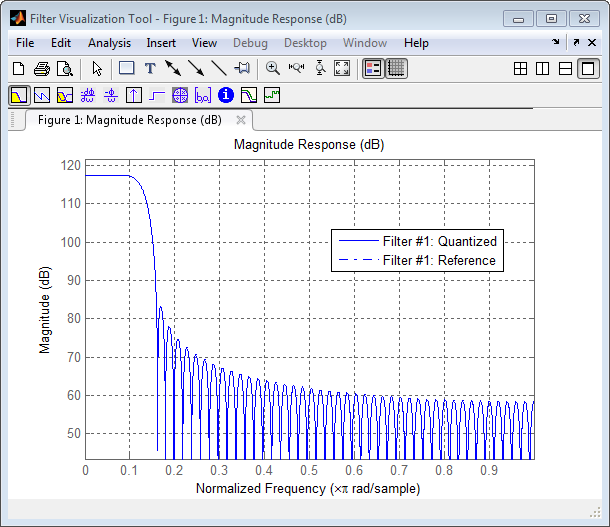This shows a large gain of 117 dB in the passband, which is due to the large values of the coefficients— this will cause the output of the filter to be much larger than the input. A method of addressing this will be discussed in the following sections.

### Set the Filter Parameters to Work with Integers

You will need to set the input parameters of your filter to appropriate values for working with integers. For example, if the input to the filter is from a A/D converter with 12 bit resolution, you should set the input as follows:

```h.InputWordLength = 12; h.InputFracLength = 0;```

The `info` method returns a summary of the filter settings.

`info(h)`

```Discrete-Time FIR Filter (real) ------------------------------- Filter Structure : Direct-Form FIR Filter Length : 101 Stable : Yes Linear Phase : Yes (Type 1) Arithmetic : fixed Numerator : s18,0 -> [-131072 131072) Input : s12,0 -> [-2048 2048) Filter Internals : Full Precision Output : s31,0 -> [-1073741824 1073741824) (auto determined) Product : s29,0 -> [-268435456 268435456) (auto determined) Accumulator : s31,0 -> [-1073741824 1073741824) (auto determined) Round Mode : No rounding Overflow Mode : No overflow ```

In this case, all the fractional lengths are now set to zero, meaning that the filter `h` is set up to handle integers.

### Create a Test Signal for the Filter

You can generate an input signal for the filter by quantizing to 12 bits using the autoscaling feature, or you can follow the same procedure that was used for the coefficients, discussed previously. In this example, create a signal with two sinusoids:

```n = 0:999; f1 = 0.1*pi; % Normalized frequency of first sinusoid f2 = 0.8*pi; % Normalized frequency of second sinusoid x = 0.9*sin(0.1*pi*n) + 0.9*sin(0.8*pi*n); xq = fi(x, true, 12); % signed = true, B = 12 xsc = fi(xq.int, true, 12, 0);```

### Filter the Test Signal

To filter the input signal generated above, enter the following:

`ysc = filter(h, xsc);`

Here `ysc` is a full precision output, meaning that no bits have been discarded in the computation. This makes `ysc` the best possible output you can achieve given the 12–bit input and the 18–bit coefficients. This can be verified by filtering using double-precision floating-point and comparing the results of the two filtering operations:

```hd = double(h); xd = double(xsc); yd = filter(hd, xd); norm(yd-double(ysc)) ans = 0 ```

Now you can examine the output compared to the input. This example is plotting only the last few samples to minimize the effect of transients:

```idx = 800:950; xscext = double(xsc(idx)'); gd = grpdelay(h, [f1 f2]); yidx = idx + gd(1); yscext = double(ysc(yidx)'); stem(n(idx)', [xscext, yscext]); axis([800 950 -2.5e8 2.5e8]); legend('input', 'output'); set(gcf, 'color', 'white');```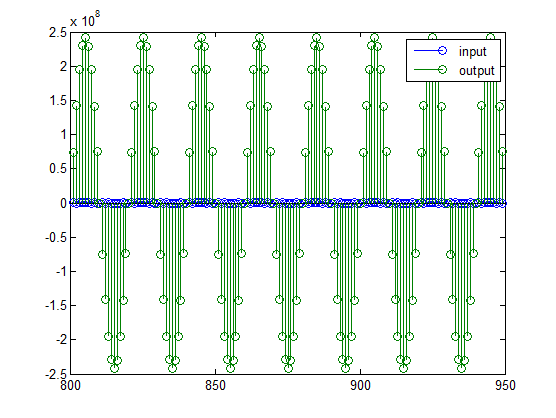It is difficult to compare the two signals in this figure because of the large difference in scales. This is due to the large gain of the filter, so you will need to compensate for the filter gain:

```stem(n(idx)', [2^18*xscext, yscext]); axis([800 950 -5e8 5e8]); legend('scaled input', 'output');```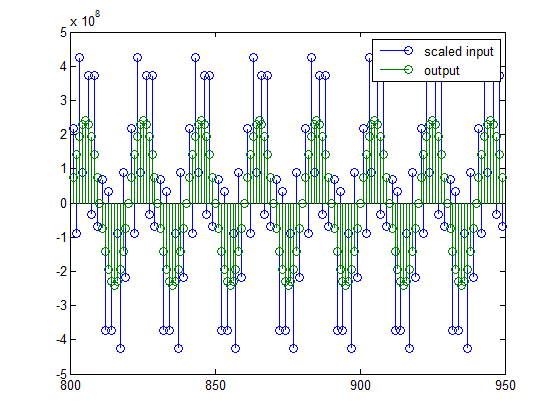You can see how the signals compare much more easily once the scaling has been done, as seen in the above figure.

### Truncate the Output WordLength

If you examine the output wordlength,

```ysc.WordLength ans = 31 ```

you will notice that the number of bits in the output is considerably greater than in the input. Because such growth in the number of bits representing the data may not be desirable, you may need to truncate the wordlength of the output. The best way to do this is to discard the least significant bits, in order to minimize error. However, if you know there are unused high order bits, you should discard those bits as well.

To determine if there are unused most significant bits (MSBs), you can look at where the growth in WordLength arises in the computation. In this case, the bit growth occurs to accommodate the results of adding products of the input (12 bits) and the coefficients (18 bits). Each of these products is 29 bits long (you can verify this using `info(h)`). The bit growth due to the accumulation of the product depends on the filter length and the coefficient values- however, this is a worst-case determination in the sense that no assumption on the input signal is made besides, and as a result there may be unused MSBs. You will have to be careful though, as MSBs that are deemed unused incorrectly will cause overflows.

Suppose you want to keep 16 bits for the output. In this case, there is no bit-growth due to the additions, so the output bit setting will be 16 for the wordlength and –14 for the fraction length.

Since the filtering has already been done, you can discard some bits from `ysc`:

`yout = fi(ysc, true, 16, -14);`

Alternatively, you can set the filter output bit lengths directly (this is useful if you plan on filtering many signals):

```specifyall(h); h.OutputWordLength = 16; h.OutputFracLength = -14; yout2 = filter(h, xsc);```

You can verify that the results are the same either way:

```norm(double(yout) - double(yout2)) ans = 0 ```

However, if you compare this to the full precision output, you will notice that there is rounding error due to the discarded bits:

```norm(double(yout)-double(ysc)) ans = 1.446323386867543e+005 ```

In this case the differences are hard to spot when plotting the data, as seen below:

```stem(n(yidx), [double(yout(yidx)'), double(ysc(yidx)')]); axis([850 950 -2.5e8 2.5e8]); legend('Scaled Input', 'Output'); set(gcf, 'color', 'white');```### Scale the Output

Because the filter in this example has such a large gain, the output is at a different scale than the input. This scaling is purely theoretical however, and you can scale the data however you like. In this case, you have 16 bits for the output, but you can attach whatever scaling you choose. It would be natural to reinterpret the output to have a weight of 2^0 (or L = 0) for the LSB. This is equivalent to scaling the output signal down by a factor of 2^(-14). However, there is no computation or rounding error involved. You can do this by executing the following:

```yri = fi(yout.int, true, 16, 0); stem(n(idx)', [xscext, double(yri(yidx)')]); axis([800 950 -1.5e4 1.5e4]); legend('input', 'rescaled output');```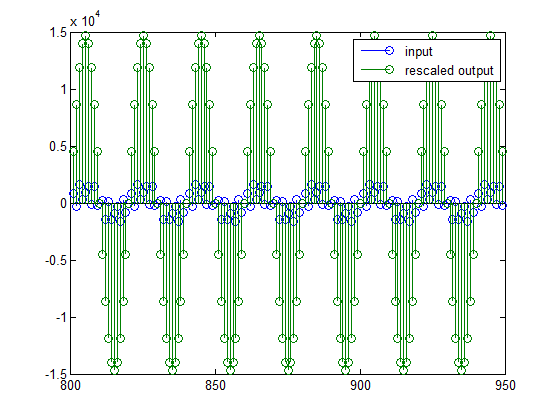This plot shows that the output is still larger than the input. If you had done the filtering in double-precision floating-point, this would not be the case— because here more bits are being used for the output than for the input, so the MSBs are weighted differently. You can see this another way by looking at the magnitude response of the scaled filter:

```[H,w] = freqz(h); plot(w/pi, 20*log10(2^(-14)*abs(H)));```This plot shows that the passband gain is still above 0 dB.

To put the input and output on the same scale, the MSBs must be weighted equally. The input MSB has a weight of 2^11, whereas the scaled output MSB has a weight of 2^(29–14) = 2^15. You need to give the output MSB a weight of 2^11 as follows:

```yf = fi(zeros(size(yri)), true, 16, 4); yf.bin = yri.bin; stem(n(idx)', [xscext, double(yf(yidx)')]); legend('input', 'rescaled output');```This operation is equivalent to scaling the filter gain down by 2^(-18).

```[H,w] = freqz(h); plot(w/pi, 20*log10(2^(-18)*abs(H)));```The above plot shows a 0 dB gain in the passband, as desired.

With this final version of the output, `yf` is no longer an integer. However this is only due to the interpretation- the integers represented by the bits in `yf` are identical to the ones represented by the bits in `yri`. You can verify this by comparing them:

```max(abs(yf.int - yri.int)) ans = 0 ```

### Configure Filter Parameters to Work with Integers Using the set2int Method

Set the Filter Parameters to Work with Integers

The `set2int` method provides a convenient way of setting filter parameters to work with integers. The method works by scaling the coefficients to integer numbers, and setting the coefficients and input fraction length to zero. This makes it possible for you to use floating-point coefficients directly.

```h = dfilt.dffir(b); h.Arithmetic = 'fixed';```

The coefficients are represented with 18 bits and the input signal is represented with 12 bits:

```g = set2int(h, 18, 12); g_dB = 20*log10(g) g_dB = 1.083707984390332e+002 ```

The `set2int` method returns the gain of the filter by scaling the coefficients to integers, so the gain is always a power of 2. You can verify that the gain we get here is consistent with the gain of the filter previously. Now you can also check that the filter `h` is set up properly to work with integers:

```info(h) Discrete-Time FIR Filter (real) ------------------------------- Filter Structure : Direct-Form FIR Filter Length : 101 Stable : Yes Linear Phase : Yes (Type 1) Arithmetic : fixed Numerator : s18,0 -> [-131072 131072) Input : s12,0 -> [-2048 2048) Filter Internals : Full Precision Output : s31,0 -> [-1073741824 1073741824) (auto determined) Product : s29,0 -> [-268435456 268435456) (auto determined) Accumulator: s31,0 -> [-1073741824 1073741824) (auto determined) Round Mode : No rounding Overflow Mode : No overflow ```

Here you can see that all fractional lengths are now set to zero, so this filter is set up properly for working with integers.

Reinterpret the Output

You can compare the output to the double-precision floating-point reference output, and verify that the computation done by the filter `h` is done in full precision.

```yint = filter(h, xsc); norm(yd - double(yint)) ans = 0 ```

You can then truncate the output to only 16 bits:

```yout = fi(yint, true, 16); stem(n(yidx), [xscext, double(yout(yidx)')]); axis([850 950 -2.5e8 2.5e8]); legend('input', 'output'); ```Once again, the plot shows that the input and output are at different scales. In order to scale the output so that the signals can be compared more easily in a plot, you will need to weigh the MSBs appropriately. You can compute the new fraction length using the gain of the filter when the coefficients were integer numbers:

```WL = yout.WordLength; FL = yout.FractionLength + log2(g); yf2 = fi(zeros(size(yout)), true, WL, FL); yf2.bin = yout.bin; stem(n(idx)', [xscext, double(yf2(yidx)')]); axis([800 950 -2e3 2e3]); legend('input', 'rescaled output');```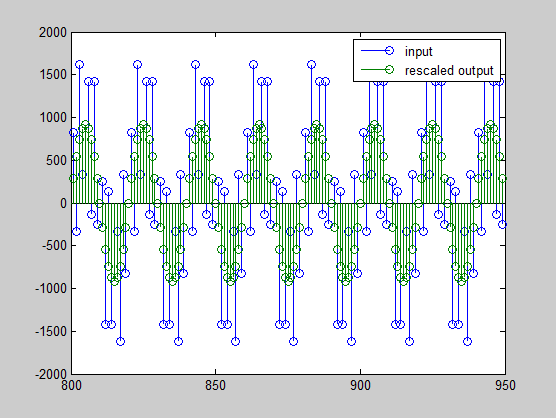This final plot shows the filtered data re-scaled to match the input scale.

## SupportGet trial now